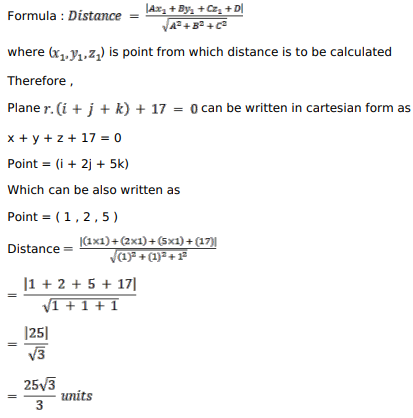# Solve this followingQuestion:

Find the distance of the point $(\hat{\mathrm{i}}+2 \hat{\mathrm{j}}+5 \hat{\mathrm{k}})$ from the plane $\overrightarrow{\mathrm{r}} \cdot(\hat{\mathrm{i}}+\hat{\mathrm{j}}+\hat{\mathrm{k}})+17=0$.

Solution: